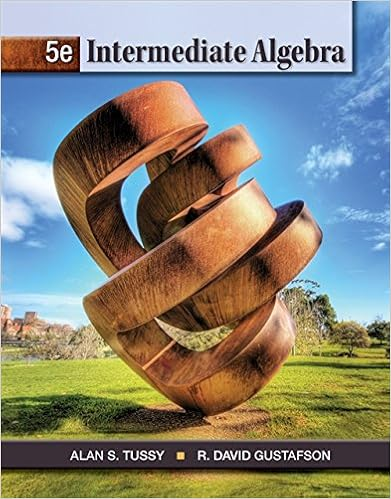# Which of the following represents the graph of 8 x 3

• Notes
• 16
• 60% (20) 12 out of 20 people found this document helpful

This preview shows page 8 - 13 out of 16 pages.

##### We have textbook solutions for you!
The document you are viewing contains questions related to this textbook.The document you are viewing contains questions related to this textbook.
Chapter 9 / Exercise 5
Intermediate Algebra
Tussy/GustafsonExpert Verified
6. Which of the following represents the graph of 8x-3y= 24 ? 6. ______ A. B. C. D.
##### We have textbook solutions for you!
The document you are viewing contains questions related to this textbook.The document you are viewing contains questions related to this textbook.
Chapter 9 / Exercise 5
Intermediate Algebra
Tussy/GustafsonExpert Verified
College Algebra MATH 107 Spring, 2012, S1.5 Page 4 of 11 7. Write a slope-intercept equation for a line parallel to the line x– 5y= 2 which passes through the point (15, –2). 7. ______
-
-
+
+8. Does the graph below represent a function and is it one-to-one? 8. ______
College Algebra MATH 107 Spring, 2012, S1.5 Page 5 of 11 9. Express as a single logarithm: log x + log 1 – 8 log y9. ______
-
10. Which of the functions corresponds to the graph? 10. ______ A. ()2xfxe= -B. ()2xfxe-=C. ()1xfxe-=D. ()2xfxe=
+
+
+
+
College Algebra MATH 107 Spring, 2012, S1.5 Page 6 of 11 11. The percentage score on a test varies directly as the number of correct responses. Al answered 27 questions correctly and earned a score of 60%. What would Al’s percentage score have been if he had answered 36 questions correctly? 11. ______
12. The graph of y= f(x) is shown at the left and the graph of y= g(x) is shown at the right. (No formulas are given.) What is the relationship between g(x) and f(x12. ______ y= f(x) y= g(2 4 -2 -2 -4 24 2 4 -2 -2 -4 24 )? x)
-4 -4
College Algebra MATH 107 Spring, 2012, S1.5 Page 7 of 11 SHORT ANSWER: 13. Multiply and simplify: (8 + 3i)(5 + 2i). Write the answer in the form a+ bi, where aand bare real numbers. Answer: ________
•••